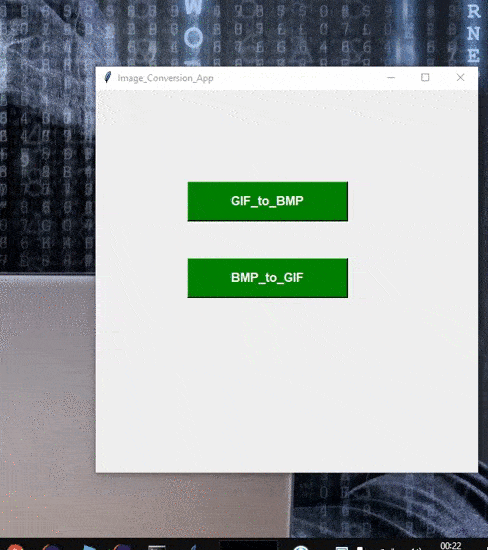# Convert the .GIF to .BMP and it’s vice-versa in Python

• Last Updated : 17 Dec, 2021

Sometimes it is required to attach the Image where we required an image file with the specified extension. And we have the image with the different extension which needs to be converted with a specified extension like in this we will convert the image having Extension of .bmp to .gif and Vice-Versa. In this article, we are going to convert .GIF to .BMP and .BMP to .GIF.

And Also we will be creating the GUI interface to the Code, so we will require the Library Tkinter. Tkinter is a Python binding to the Tk GUI toolkit. It is the standard Python interface to the Tk GUI toolkit which provides the interface to the GUI apps.

## Modules Needed:

• Tkinter: Tkinter is a Python binding to the Tk GUI toolkit.
• PIL: It is a Python Imaging Library that provides the python interpreter with image editing capabilities.

Let’s implement with step-wise:

Step 1: Import the library.

`from PIL import Image`

Step 2: JPG to GIF

```To convert the image From BMP to GIF : {Syntax}
img = Image.open("Image.bmp")
img.save("Image.gif")```

Step 3: GIF to JPG

```To convert the Image From GIF to PNG
img = Image.open("Image.gif")
img.save("Image.bmp")```

Approach:

• In Function bmp_to_gif we first Check whether The Selecting the image is in the same Format (.bmp) which to convert to .gif if not then return Error.
• Else Convert the image the to .gif
• To open the Image we use the Function in tkinter called the FileDialog which helps to open the image from the folder
• from tkinter import filedialog as fd
• Same Approach for the GIF to BMP

Below is the full implementation:

## Python3

 `from` `tkinter ``import` `*``from` `tkinter ``import` `filedialog as fd``import` `os``from` `PIL ``import` `Image``from` `tkinter ``import` `messagebox``  ` `root ``=` `Tk()``  ` `# naming the GUI interface to image_conversion_APP``root.title(``"Image_Conversion_App"``)``  ` `# creating the Function which converts the jpg_to_png``def` `gif_to_bmp():``    ``global` `im``    ` `    ``import_filename ``=` `fd.askopenfilename()``    ` `    ``if` `import_filename.endswith(``".gif"``):``      ` `        ``im ``=` `Image.``open``(import_filename)``        ``export_filename ``=` `fd.asksaveasfilename(defaultextension ``=` `".bmp"``)``        ``im.save(export_filename)``        ``messagebox.showinfo(``"Success"``, ``"File converted to .png"``)``    ``else``:``        ``messagebox.showerror(``"Fail!!"``, ``"Error Interrupted!!!! Check Again"``)``def` `bmp_to_gif():``  ` `    ``import_filename ``=` `fd.askopenfilename()``    ``if` `import_filename.endswith(``".bmp"``):``      ` `        ``im ``=` `Image.``open``(import_filename)``        ``export_filename ``=` `fd.asksaveasfilename(defaultextension ``=` `".gif"``)``        ``im.save(export_filename)``        ``messagebox.showinfo(``"Success"``, ``"File converted to .gif"``)``    ``else``:``      ` `        ``messagebox.showerror(``"Fail!!"``, ``"Error Interrupted!!!! Check Again"``)` `button1 ``=` `Button(root, text ``=` `"GIF_to_BMP"``,``                 ``width ``=` `20``, height ``=` `2``,``                 ``bg ``=` `"green"``, fg ``=` `"white"``,``                 ``font ``=` `(``"helvetica"``, ``12``, ``"bold"``),``                 ``command ``=` `gif_to_bmp)``  ` `button1.place(x ``=` `120``, y ``=` `120``)``  ` `button2 ``=` `Button(root, text ``=` `"BMP_to_GIF"``,``                 ``width ``=` `20``, height ``=` `2``,``                 ``bg ``=` `"green"``, fg ``=` `"white"``,``                 ``font ``=` `(``"helvetica"``, ``12``, ``"bold"``),``                 ``command ``=` `bmp_to_gif)``  ` `button2.place(x ``=` `120``, y ``=` `220``)``root.geometry(``"500x500+400+200"``)``root.mainloop()`

Output:My Personal Notes arrow_drop_up